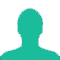# Find the number of triangles which can be formed by joining the angular points of a polygon of 8 sides as vertices.

A. 56

B. 24

C. 16

D. 8

### Solution(By Examveda Team)

A triangle needs 3 points.
And polygon of 8 sides has 8 angular points.
Hence, number of triangle formed,
\eqalign{ & { = ^8}{{\text{C}}_3} \cr & = \frac{{8 \times 7 \times 6}}{{1 \times 2 \times 3}} \cr & = 56 \cr}

1.